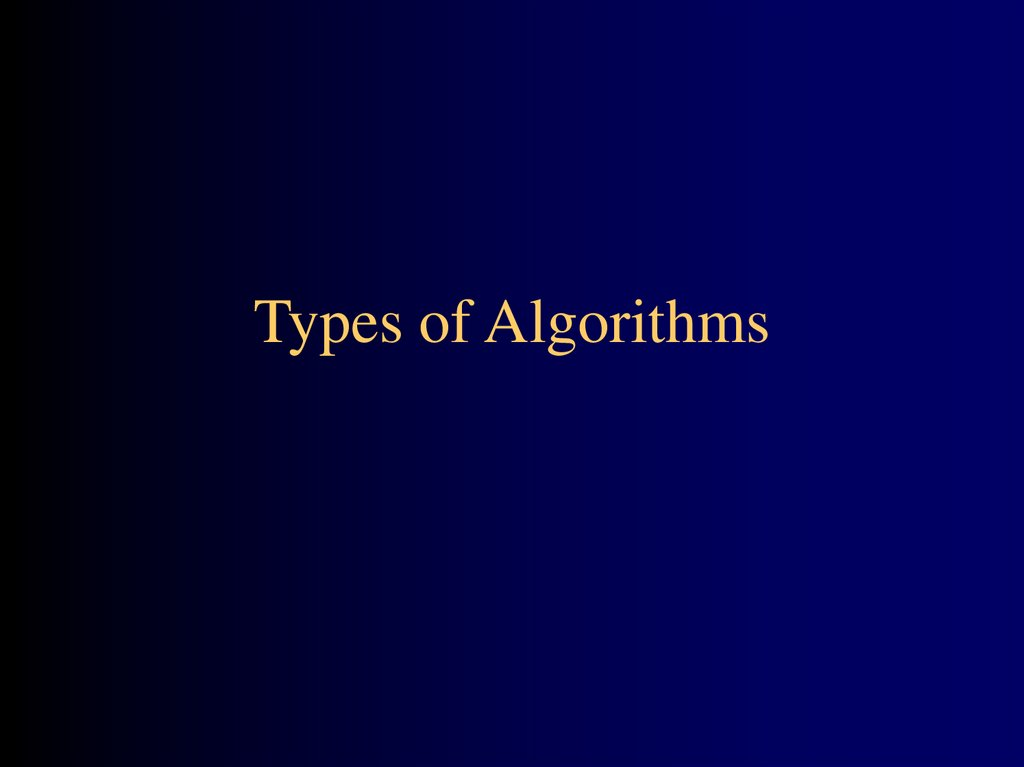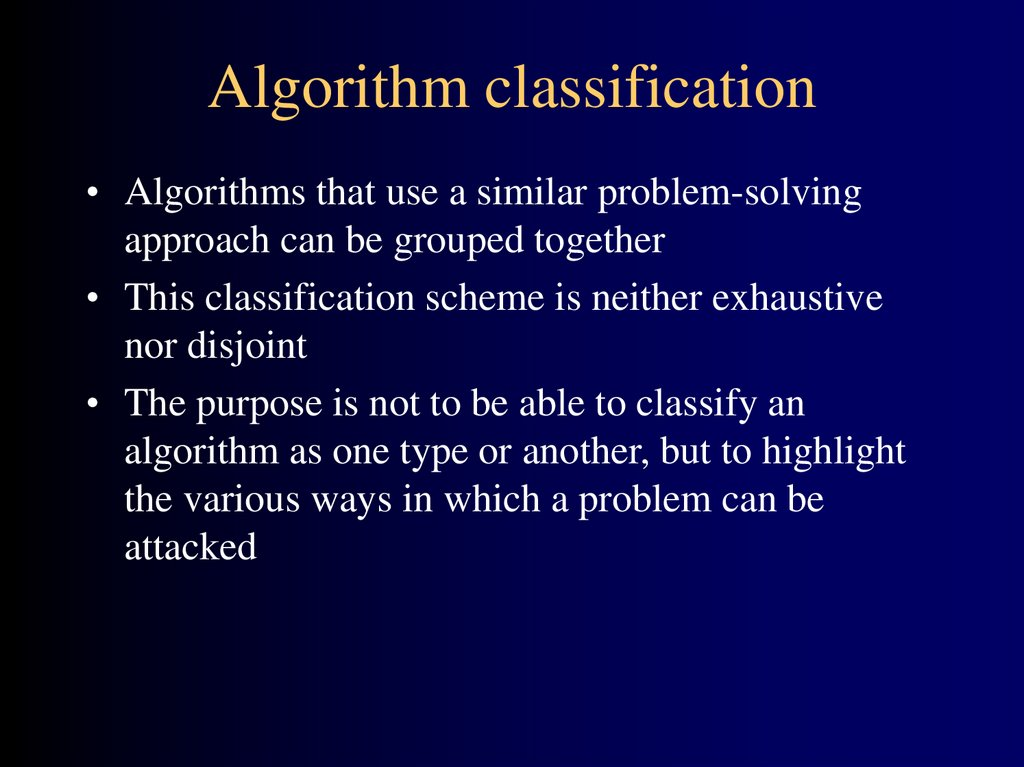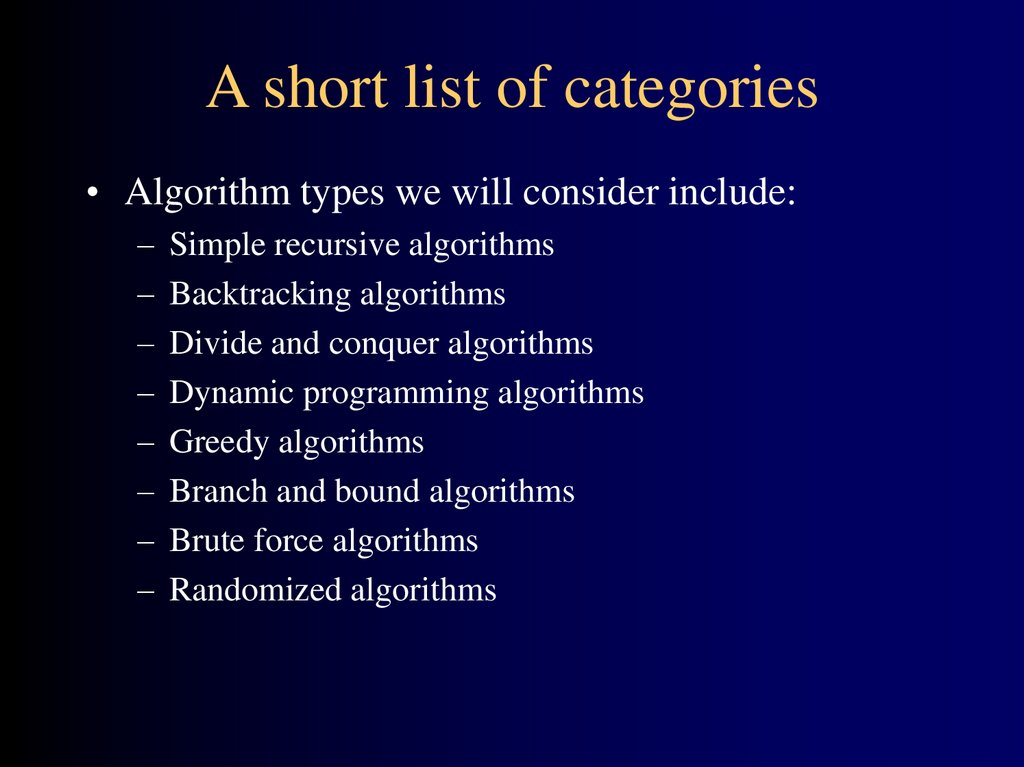# Types of algorithms

## 2. Algorithm classification

• Algorithms that use a similar problem-solving
approach can be grouped together
• This classification scheme is neither exhaustive
nor disjoint
• The purpose is not to be able to classify an
algorithm as one type or another, but to highlight
the various ways in which a problem can be
attacked

## 3. A short list of categories

• Algorithm types we will consider include:

Simple recursive algorithms
Backtracking algorithms
Divide and conquer algorithms
Dynamic programming algorithms
Greedy algorithms
Branch and bound algorithms
Brute force algorithms
Randomized algorithms

## 4. Simple recursive algorithms I

• A simple recursive algorithm:
– Solves the base cases directly
– Recurs with a simpler subproblem
– Does some extra work to convert the solution to the
simpler subproblem into a solution to the given
problem
• I call these “simple” because several of the other
algorithm types are inherently recursive

## 5. Example recursive algorithms

• To count the number of elements in a list:
– If the list is empty, return zero; otherwise,
– Step past the first element, and count the remaining
elements in the list
– Add one to the result
• To test if a value occurs in a list:
– If the list is empty, return false; otherwise,
– If the first thing in the list is the given value, return
true; otherwise
– Step past the first element, and test whether the value
occurs in the remainder of the list

## 6. Backtracking algorithms

• Backtracking algorithms are based on a depth-first
recursive search
• A backtracking algorithm:
– Tests to see if a solution has been found, and if so,
returns it; otherwise
– For each choice that can be made at this point,
• Make that choice
• Recur
• If the recursion returns a solution, return it
– If no choices remain, return failure

## 7. Example backtracking algorithm

• To color a map with no more than four colors:
– color(Country n)
• If all countries have been colored (n > number of
countries) return success; otherwise,
• For each color c of four colors,
– If country n is not adjacent to a country that has
been colored c
» Color country n with color c
» recursivly color country n+1
» If successful, return success
• Return failure (if loop exits)

## 8. Divide and Conquer

A divide and conquer algorithm consists of two
parts:
– Divide the problem into smaller subproblems of the
same type, and solve these subproblems recursively
– Combine the solutions to the subproblems into a
solution to the original problem
Traditionally, an algorithm is only called divide
and conquer if it contains two or more recursive
calls

## 9. Examples

• Quicksort:
– Partition the array into two parts, and quicksort each
of the parts
– No additional work is required to combine the two
sorted parts
• Mergesort:
– Cut the array in half, and mergesort each half
– Combine the two sorted arrays into a single sorted
array by merging them

## 10. Binary tree lookup

• Here’s how to look up something in a sorted
binary tree:
– Compare the key to the value in the root
• If the two values are equal, report success
• If the key is less, search the left subtree
• If the key is greater, search the right subtree
• This is not a divide and conquer algorithm
because, although there are two recursive calls,
only one is used at each level of the recursion

## 11. Fibonacci numbers

• To find the nth Fibonacci number:
– If n is zero or one, return one; otherwise,
– Compute fibonacci(n-1) and fibonacci(n-2)
– Return the sum of these two numbers
• This is an expensive algorithm
– It requires O(fibonacci(n)) time
– This is equivalent to exponential time, that is, O(2n)

## 12. Dynamic programming algorithms

• A dynamic programming algorithm remembers past results
and uses them to find new results
• Dynamic programming is generally used for optimization
problems
– Multiple solutions exist, need to find the “best” one
– Requires “optimal substructure” and “overlapping subproblems”
• Optimal substructure: Optimal solution contains optimal
solutions to subproblems
• Overlapping subproblems: Solutions to subproblems can be
stored and reused in a bottom-up fashion
• This differs from Divide and Conquer, where subproblems
generally need not overlap

## 13. Fibonacci numbers again

• To find the nth Fibonacci number:
– If n is zero or one, return one; otherwise,
– Compute, or look up in a table, fibonacci(n-1) and
fibonacci(n-2)
– Find the sum of these two numbers
– Store the result in a table and return it
• Since finding the nth Fibonacci number involves
finding all smaller Fibonacci numbers, the second
recursive call has little work to do
• The table may be preserved and used again later

## 14. Greedy algorithms

• An optimization problem is one in which you want
to find, not just a solution, but the best solution
• A “greedy algorithm” sometimes works well for
optimization problems
• A greedy algorithm works in phases: At each
phase:
– You take the best you can get right now, without regard
for future consequences
– You hope that by choosing a local optimum at each
step, you will end up at a global optimum

## 15. Example: Counting money

• Suppose you want to count out a certain amount of money,
using the fewest possible bills and coins
• A greedy algorithm would do this would be:
At each step, take the largest possible bill or coin that does
not overshoot
– Example: To make \$6.39, you can choose:
• a \$5 bill
• a \$1 bill, to make \$6
• a 25¢ coin, to make \$6.25
• A 10¢ coin, to make \$6.35
• four 1¢ coins, to make \$6.39
• For US money, the greedy algorithm always gives the
optimum solution

## 16. A failure of the greedy algorithm

• In some (fictional) monetary system, “krons” come
in 1 kron, 7 kron, and 10 kron coins
• Using a greedy algorithm to count out 15 krons,
you would get
– A 10 kron piece
– Five 1 kron pieces, for a total of 15 krons
– This requires six coins
• A better solution would be to use two 7 kron pieces
and one 1 kron piece
– This only requires three coins
• The greedy algorithm results in a solution, but not
in an optimal solution

## 17. Branch and bound algorithms

• Branch and bound algorithms are generally used for
optimization problems
– As the algorithm progresses, a tree of subproblems is formed
– The original problem is considered the “root problem”
– A method is used to construct an upper and lower bound for a
given problem
– At each node, apply the bounding methods
• If the bounds match, it is deemed a feasible solution to that
particular subproblem
• If bounds do not match, partition the problem represented by
that node, and make the two subproblems into children nodes
– Continue, using the best known feasible solution to trim sections of
the tree, until all nodes have been solved or trimmed

## 18. Example branch and bound algorithm

• Travelling salesman problem: A salesman has to
visit each of n cities (at least) once each, and
wants to minimize total distance travelled
– Consider the root problem to be the problem of finding
the shortest route through a set of cities visiting each
city once
– Split the node into two child problems:
• Shortest route visiting city A first
• Shortest route not visiting city A first
– Continue subdividing similarly as the tree grows

## 19. Brute force algorithm

• A brute force algorithm simply tries all
possibilities until a satisfactory solution is found
– Such an algorithm can be:
• Optimizing: Find the best solution. This may require
finding all solutions, or if a value for the best
solution is known, it may stop when any best
solution is found
– Example: Finding the best path for a travelling salesman
• Satisficing: Stop as soon as a solution is found that
is good enough
– Example: Finding a travelling salesman path that is within
10% of optimal

## 20. Improving brute force algorithms

• Often, brute force algorithms require exponential
time
• Various heuristics and optimizations can be used
– Heuristic: A “rule of thumb” that helps you decide
which possibilities to look at first
– Optimization: In this case, a way to eliminate certain
possibilites without fully exploring them

## 21. Randomized algorithms

• A randomized algorithm uses a random number at
least once during the computation to make a
decision
– Example: In Quicksort, using a random number to
choose a pivot
– Example: Trying to factor a large prime by choosing
random numbers as possible divisors